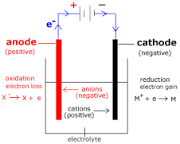# ELECTROLYSIS

Electrolysis is a technique that uses a direct electric current (DC) to drive an otherwise non-spontaneous chemical reaction. Electrolysis is also important as a stage in the separation of elements from naturally occurring sources such as ores using an electrolytic cell.

It also refers to the decomposition of a substance by an electric current. The electrolysis of sodium and potassium hydroxides, first carried out in 1808 by Sir Humphrey Davey, led to the discovery of these two metallic elements and showed that these two hydroxides which had previously been considered un-decomposable and thus elements, were in fact compounds

Electrolysis of molten alkali halides is the usual industrial method of preparing the alkali metals:

 cathode: Na+ + e– → Na(l) E° = –2.71 v anode: Cl– → ½ Cl2(g) + e– E° = –1.36 v net: Na+ + Cl– → Na(l) + ½ Cl2(g) E° = –4.1 v

Ions in aqueous solutions can undergo similar reactions. Thus if a solution of nickel chloride undergoes electrolysis at platinum electrodes, the reactions are

 cathode: Ni2+ + 2 e– → Ni(s) E° = –0.24 v anode: 2 Cl– → Cl2(g) + 2 e– E° = –1.36 v net: Ni2+ + 2 Cl– → Ni(s) + Cl2(g) E° = –1.60 vFARADAY’S LAWS OF ELECTROLYSIS

First law of electrolysis

In 1832, Michael Faraday reported that the quantity of elements separated by passing an electric current through a molten or dissolved salt is proportional to the quantity of electric charge passed through the circuit. This became the basis of the first law of electrolysis:$m = k \cdot q$

or$m = eQ$

where; e is known as electrochemical equivalent of the metal deposited or of the gas liberated at the electrode.

Second law of electrolysis

Faraday discovered that when the same amount of current is passed through different electrolytes/elements connected in series, the mass of substance liberated/deposited at the electrodes is directly proportional to their equivalent weight.

WHAT HAPPENS TO COPPER DURING ELECTROLYSIS:

Positively charged ions move to the negative electrode during electrolysis. They receive electrons and are reduced. Negatively charged ions move to the positive electrode during electrolysis.

PROBLEMS AND SOLUTIONS ON ELECTROLYSIS:

PROBLEM 1.

A metallic object to be plated with copper is placed in a solution of CuSO4.

a) To which electrode of a direct current power supply should the object be connected?
b) What mass of copper will be deposited if a current of 0.22 amp flows through the cell for 1.5 hours?

Solution:

a) Since Cu2+ ions are being reduced, the object acts as a cathode and must be connected to the negative terminal (where the electrons come from!)

b) The amount of charge passing through the cell is

(0.22 amp) × (5400 sec) = 1200 c
or
(1200 c) ÷ (96500 c F–1) = 0.012 F

Since the reduction of one mole of Cu2+ ion requires the addition of two moles of electrons, the mass of Cu deposited will be

(63.54 g mol–1) (0.5 mol Cu/F) (.012 F) = 0.39 g of copper.

PROBLEM 2.

How long must a 20.0 amp current flow through a solution of ZnSO4 in order to produce 25.00 g of Zn metal.

Solution:

• Convert the mass of Zn produced into moles using the molar mass of Zn.

Mass of Zn = 25.00g, molarmass of Zn = 65gmol-1

Mole = mass/molarmass

Mole = 25/65 = 0.3846mol

• Write the half-reaction for the production of Zn at the cathode.

Zn2+(aq) + 2e  Zn(s)

• Calculate the moles of e required to produce the moles of Zn and convert the moles of electrons into coulombs of charge using Faraday’s constant.

1 mole of Zn liberate 2F (2 x 96500)C

0.386 mole will liberate: (2 x 96500 x 0.3846/1) = 74231C

• Calculate the time using the current and the coulombs of charge.

Q = I x t

t = Q / I

t = 74231/20

t = 3712secs = 62 minutes = 1.03hr

For more information visit ‘ask a question page’ on http://www.basic2tech.wordpress.com and like our page on

Facebook @ basic2tech online classroom